# Design Of Retaining WallsDesign of cantilever and counter fort retaining walls : Retaining wall - Retains Earth - when level difference exists between two surfaces

Retaining wall -Retains Earth -when level difference exists between two surfaces

A)  Gravity wall (h<3m) -Masonry or Stone

B)  Cantilever wall (h>3m and h<6m)

C)  Counterfort wall (h>8m)

D)   Buttress wall [Transverse stem support provided on front side]

E)E) Bridge abutment [Additional horizontal restraint from bridge deck]

A)  Gravity wall (h<3m) -Masonry or Stone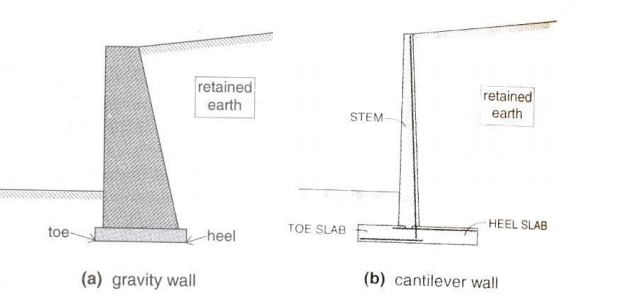B)  Cantilever wall (h>3m and h<6m)

C)  Counterfort wall (h>8m)D)   Buttress wall [Transverse stem support provided on front side]E)E) Bridge abutment [Additional horizontal restraint from bridge deck]

Stability -Overturning and Sliding -Avoided by providing sufficient base width.

Earth pressure and stability requirements:Pressure, P =CgZ

Where, Z = depth, g = Unit weight

Ca = 1 -Sinf  / 1 +Sinf

Cp = 1 +Sinf / 1 -Sinf

Always, Cp > Ca.

Eg:   If0, Ca?= 1/3=and30Cp = 3.

In sloped backfill,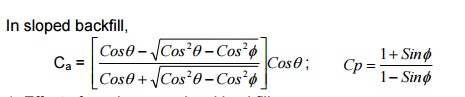1.Effect of surcharge on level backfill:Pa = Pa1 + Pa2, where,

Note : Purpose of retaining wall is to retain earth and not water. Therefore, submerged condition should be avoided by providing and maintaining proper drainage facilities [including provision of weep holes].

2. Effect of water in the backfill:Stability requirementsii) Sliding: [Friction between base slab and supporting soil]

F    =   ?.[where,R R = W]

R -> Resultant soil pressure at footing base

?-> Coefficient of static friction [0.35 -Silt & 0.60 -Rough rock]

FOSsliding = 0.9F /  Pa Cosq ?   1.4

When Pa is very high, shear key projection can be provided below footing base [Produces passive resistance Pps, which is generally neglected, otherwise].

Sliding is reduced by providing shear key [like a plug, anchors inside]Pps  =Cge(h2 2  -h12 ) / 2

Xsk -> Flexural reinforcement from stem is extended straight into shear key near the

toe.

Note: For economical design, soil pressure resultant(R) must be in line with front face of wall.

Preliminary proportioning of cantilever retaining wall:

1.   The thickness of base slab is h/12 or 8% of the height of wall + surcharge.

2.   The base thickness of stem should be greater than the thickness of base slab

3.   The top thickness of stem should not be less than 150mm.

4. Clear cover for stem  is 50mm and base slab is 75mm

5. Minimum length of base slab is given by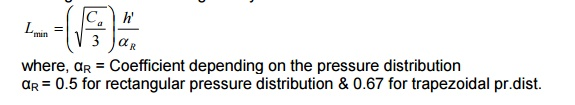where,R=Coefficient? depending on the pressure distribution

?R = 0.5 for rectangular pressure distribution & 0.67 for trapezoidal pr.dist.

6.   Minimum length of heel slab is given by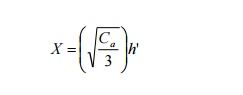Notes:

1.   The critical section for moment is at front face of stem.

2.   The   critical   section   for  shear   is   at   �d?  f

3.   The stem, heel and toe slabs are designed as cantilever slabs for the resultant pressure.

4.   Temperature and shrinkage reinforcement is provided as 0.12% of cross section along the transverse direction to the main reinforcement and front face of the stem.

1. Determine suitable dimensions of a cantilever retaining wall, which is required to support a bank of earth 4.0m high above ground level on the toe side of the wall. Consider the backfill surface to be inclined at an angle of 15o with the horizontal. Assume good soil for foundation at a depth of 1.25m below ground level with SBC of 160kN/m2. Further assume the backfill to comprise of granular soil with unit weight of 16kN/m3 and an angle of shearing resistance of 30o. Assume the coefficient of friction between soil and concrete to be 0.5.

Given: h = 4.0 + 1.25m

? =o 15 ? = o 30

re = 16kN/m3

qa = 160kN/m2

?      =   0.5

Minimum   depth   of   foundation   (Rankine?s),Preliminary proportioning:

Thickness of footing base slab = 0.08h = 0.08 x 5.25 = 0.42m

Provide a base thickness of 420mm for base slab.

Assume stem thickness of 450mm at base of stem tapering to 150mm at top of wall.

For economical proportioning of length �L?, a be in line with front face of the stem.

height above wall]Assuming a triangular base pressure distribution, L = 1.5X = 3.0m

Preliminary proportions are shown in figure.

For the assumed proportions, the retaining wall is checked for stability against overturning and sliding.

Stability against overturning:Pa = Active pressure exerted by retained earth on wall [both wall and earth move in same direction]

Pp = Passive pressure exerted by wall on retained earth [both move in opposite direction]

Ca -> same for dry and submerged condition, si significantly.

Force due to active pressure,Pa = 0.373(16)(5.786)2/2 = 99.9kN                                                         [per m length of wall]

FOS =  0.9   x   Stabilising   force   or  moment / Destabilising force or moment

Therefore, FOS(overturning) = 0.9Mr / Mo ? 1.4

Overturning moment, Mo = (PaCos?)h?/3   =   96.5(5.786/3)   =   186.

To find the distance of resultant vertical force from heel,

Distance of resultant vertical force from heel,

Xw = Mw/W = 230.6/232.9 = 0.99m

Mr = W(L -Xw) + PaSin?(L) =-0 .99)232+77.69(3 = 468.1kNm [per m length of wall]

FOS(overturning) = 0.9Mr / Mo = 0.9x(468.1 +77.6) / 186.1 = 2.26 > 1.40

Soil pressure at footing base:

Resultant vertical reaction, R = W = 232.9kN   [per m length of wall]Distance of R from heel, LR = (Mw + Mo) / R = (230.6 + 186.1)/232.9 = 1.789m

Eccentricity, e = LR -L/2 = 1.789 -3/2 = 0.289m < L/6 ->[0.5m]

Hence the resultant lies within the middle third of the base, which is desirable.

Maximum pressure as base,Stability against sliding:

Sliding force, PaCos?   =   96.5kN

Resisting     force,   F   =   ?R  =[Ignoring0 .passive5 pressurex232ontoe.side]9   =  116.4

FOS(Sliding) = 0.9F / Pa Cosq  = 0.9x116.4 / 96.5 = 1.085 <1.40 [Not sufficient]

Hence a shear key may be provided.

Assume a shear key 300mm x 300mm at a distance of 1300mm from toe as shown in figure.h2     = 950 + 300 + 1300tan30o  = 2001mm

Pp = Cp.e?.(h22 -h12)/2 = 3 x 16 x (2.0012 -0.9502)/2 = 74.11kN.

FOS (Sliding) = 0.9(116.4 +74.44) / 96.5 =1.78 >1.4 [SAFE]

Design of toe slab:

Assuming a   clear   cover   of   75mm   and   16mm   ?   use

d = 420 -75 -8 = 337mm

Vu = 1.5 [112 +81.9  / 2] x(1 - 0.337)  = 96.42kN [Vu is   design  shear   at   �d?   from   fac

Mu = 1.5{(81.9 x 12/2) + (112 - 81.9) x 1/2 x 12 x

2/3} = 76.48 kNm/m length

Nominal shear stress, t = V/ bd = 96.42x103 / 1000x337  =0.286N / mm2

Using M20 concrete,

For a t =0.29N/mm2, pt (required) = 0.2%

K = M u bd 2 = 76.48x106  / 1000x3372 =0.673N / mm2

For pt = 0.2%, Ast = 0.2/100 x 1000 x 337 = 674 mm2 / m

Spacing = ( 1000xpx162  / 4 ) /  674 = 298mm

Provide 16mmf @ 290mm c/c at bottom of toe slab

Ld = fs s  / 4t bd  =  (16).0.87 f y / 4t bd  = 752mm, beyond face of stem.

Since length available is 1m, no curtailment is sorted.

Design of heel slab:

Vu = =128.06kN

Mu = 1.5{(82.54 x 1.552/2) + (128.6 - 82.54) x 1/2 x 1.552 x

2/3} = 203.96 kNm/m length

Nominal shear stress, t = V / bd = 128.06x103  / 1000x337 = =0.38N / mm2

Using M20 concrete,

For a t =0.39N/mm2, pt (required) = 0.3%

K = M u / bd 2  = 203.96x106 / 1000x3372 = 1.8N / mm2

For pt = 0.565%, Ast = 0.565/100 x 1000 x 337 = 1904.05 mm2 / m

Spacing = (1000xpx162  / 4) / 1904.05 = 105.61mm

Provide 16mmf @ 100mm c/c at bottom of toe slab

Ld = fs s / 4t bd = (16).0.87 f y / 4tbd = 16 x 47 = 752mm, beyond face of stem.

Since length available is 1.55m, no curtailment is sorted.

Design of vertical stem:

Height of cantilever above base = 5250 -420 = 4830mm

Assume a clear cover of 50mm and 16mm? bar, dat base = 450 -50 -16/2 = 392mm

Mu = 1.5(Ca.e?.h3/6) = 1.5(1/3)(16 x 4.923/6) = 150.24kNm.

K = Mu / bd2 = 150.24x106  / 1000x4.922  = 1N/mm2

pt = 0.3%, Ast = 0.295/100 x 1000 x 392 = 1200mm2. Spacing = 1000 x 201/1200 = 160mm

Provide 16mmf @   160mm   c/c   in   the   stem,   extending=   int

752mm.

Check for shear:                                                                                                [at   �d?  from   base]

Vu (stem) = 1.5(Ca.e?.Z2/2) = 1.5(1/3 x 16 x (4.83 -0.392)2/2) = 53.83kN

tv= Vu / bd = 53.83x103 / 1000x392 = =0.135N / mm2  <tc

[where,c=0.39N/mm?2 for pt = 0.3%))

Hence, SAFE.

Curtailment of bars:

Curtailments of bars in stem are done in two stages:

At 1/3rd and 2/3rd height of the stem above base.

Temperature and shrinkage reinforcement:

Ast = 0.12/100 x 10 x 450 = 540mm2

For I 1/3rd height, provide 2/3rd of bar near front face (exposed to weather) and 1/3rd near rear face.

For II 1/3rd height, provide 1/2 the above. For III 1/3rd height, provide 1/3rd of I case.

Provide nominal bars of 10mm @ 300mm c/c vertically near front face.DESIGN OF COUNTERFORT RETAINING WALLPreliminary proportioning of counterfort retaining wall:

1.   Thickness of heel slab and stem = 5% of Height of wall

2.   Thickness of toe slab [buttress not provided] = 8% of Height of wall

3.   Thickness of counterfort = 6% of height of wall

4.   In no case thickness of any component be less than 300mm.

5.   Spacing of counterforts = 1/3rd to 1/2  of Height of wall

6.   Each panel of stem and heel slab are designed as two way slab with one edge free (one way continuous slab).

7.   The toe slab is designed as

a.   Cantilever slab when buttress is not provided

b.   One way continuous slab, when buttress is provided

8.   Counterfort is a triangular shaped structure designed similar to a T-Beam as vertical cantilever with varying depth (stem acts as flange). The main reinforcement is along the sloping side. Stirrups are provided in the counterfort to secure them firmly with the stem. Additional ties are provided to securely tie the counterfort to the heel slab.

1) Design a suitable counterfort retaining wall to support a leveled backfill of height 7.5m above ground level on the toe side. Assume good soil for the foundation at a depth of 1.5m below ground level. The SBC of soil is 170kN/m2 with unit weight as 16kN/m3. The angle  of internal friction.Thecoefficientof isfriction ?between=30thesoil and concrete is 0.5. Use M25 concrete and Fe415 steel.Depth of foundation = 1.5m

Height of wall = 7.5 + 1.5 = 9m

Thickness              of   heel   and   stem   =  5%   of   9m   =   0.45m

Thickness of toe slab = 8% of 9m = 0.72m

Lmin = 1.5 x 3 = 4.5m

Thickness of counterfort = 6% of 9 = 0.54m

Stability Condition:

Earth pressure calculations:Xw = 874.69/510.75 = 1.713m

FOS (overturning) = 0.9Mr/Mo

Where

Mo = Pa.h/3 = Ca.e?.h3/6 = 0.33x16x93/6 = 647.35kNm.

Mr = (L -Xw).W = 510.75(4.5 -1.713) = 1423.6kNm.

FOS (overturning) = 1.98 > 1.4

Hence, section is safe against overturning.

Sliding:

FOS (sliding) =   0.9(?R)/PaCos?

F      =   ?R   =   0.5   x  510.75   =   255.375kN

Pa = Ca.e?.h2/2 = 215.784Where, LR = (M + Mo)/R, e = LR -L/2, where, LR = (874.688 + 647.352)/510.75 = 2.98m

& e = LR -L/2 = 2.98 -(4.5/2) = 0.73 < L/6  (0.75m)

Since the maximum earth pressure is greater than SBC of soil, the length of base slab has to be increased preferably along the toe side. Increase the toe slab by 0.5m in length.

?W  =  510.75   +   0.5   x  25   x  0.72   =  519.75kNAdditional load due to increase in toe slab by 0.5m is, Moment = 0.5/2 + 4.5 = 4.75m

?M   =   874.69   +   42.75  =   917.438kNm.

LR = (Mo + M) / R = (917.438 + 647.352)/519.75 = 3.011m

e= LR -L/2 = 3.011 -(5/2) = 0.511m < L/6 - > (0.833m)FOS (Sliding) =   0.9(?R)/Pa=0.9(0.5x519.75)/215.784 = 1.08 < 1.4.

Hence the section is not safe against sliding. Shear key is provided to resist sliding. Assume shear key of size 300 x 300mm.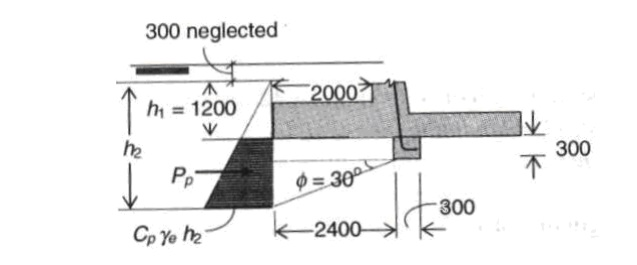Pps = Cp.e?.(h22 -h12)/2 = 164.5kN/m

FOS (sliding) =   0.9(?Rps)/Pa = +1.77P> 1.4       [where, h1 = 1.2m, h2 = 1.2 + 0.3 + 1.39 = 2.88m]

Hence, section is safe in sliding with shear key 300 x 300mm.Design of Toe Slab:

Effective cover = 75 + 20/2 = 85mm

Toe slab is designed similar to cantilever slab with maximum moment at front face of the stem and maximum shear at�d?fromfront face of stem.

d = 720 -85 = 635mm.

M = 80.38x22/2 + � x 2 x 49.94 x 2/3 x 2 = 160.76 + 122.76 = 227.35kNm.

SF at 0.635m, = 49.94/2 x 0.635 = 15.606kN

Area of trapezoid = �.h.(a + b) = �(2 -0.635)(130.32 + 95.98) = 154.44kN

Factored SF = 231.66kN; Factored Moment = 341.02kNm.

K = Mu/bd2 Ast = 1551.25mm2 Spacing = 1000ast/Ast 16mm @125mmc/c.

Transverse reinforcement: = 0.12% of c/s

= 0.12/100 x 1000 x 720 = 864mm2

Provide 10mm @100mm c/c.

Design of heel slab:

The heel slab is designed as an one way continuous slab with moment wl2/12 at the support and wl2/16 at the midspan. The maximum shear at the support is w(l/2 -d). The maximum pressure at the heel slab is considered for the design.

Moment at the support, Msup = wl2/12 = 106.92 x 2.52/12 = 55.688kNm.

Moment at the midspan, Mmid = wl2/16 = 41.76kNm

The maximum   pressure   acting   on   the   heelstrequiredslabat

midspan and support are found.

Factored Msup = 83.53kNm Ast = 570.7mm2

Factored Mmid = 62.64kNm Ast = 425.4mm2

Using   16mm   ?   bar,   Spacing  =Provide1000ast16mm@110mm/Astc/c

At midspan, spacing = 156.72mm Provide 16mm @ 150mm c/c

Transverse reinforcement = 0.12% of c/s = 0.12/100 x 1000 x 500 = 600mm2

For 8mm bar, Spacing = 83.775mm  Provide 8mm @80mm c/c.

Check for shear:

Maximum shear = w (l/2 -d) = 107 (2.5/2 -0.415) = 89.345kN

Factored shear force = 134.0175kN

?v = 0.33N/mm ,   ?c   =  0,.29N/mm?cmax  =   3.1N/mm

Depth has to be increased. Design of stem:

The stem is also designed as one way continuous slab with support moment wl2/12 and midspan moment wl2/16. For the negative moment at the support, reinforcement is provided at the rear side and for positive moment at midspan, reinforcement is provided at front face of the stem.

The maximum moment varies from a base intensity of Ka.e?.h=1/3x16x(9-0.5)= 45.33kN/m Msup = wl2/12 = 1.5 x 45.33 x 3.542/12 = 71kNm

Mmid = wl2/16 = 1.5 x 45.33 x 3.542/16 = 53.26kNm Effective depth = 500 -(50 + 20/2) = 440mm

Ast at support = 1058mm2,   For  16mm   ?,190mmSpacing.Provide16mm =@ 190mm c/c

Ast at midspan =718mm2, For 16mm ?,280mmSpacing.Provide16mm@280mm= c/c Max. SF = w (l/2 -d) = 60.29kN, Factored SF = 90.44kN

Transverse reinforcement = 0.12% of c/s 8mm @ 80mmc/c

?v = 0.188N/mm2,c =?0.65N/mm2,cmax?= 3.1N/mm2 Safe in Shear. Design of Counterfort:

The counterfort is designed as a cantilever beam whose depth is equal to the length of the heel slab at the base and reduces to the thickness of the stem at the top. Maximum moment at the base of counterfort, Mmax = Ka.e?.h3/6 x Le

Where, Le c/c distance from counterfort

Mmax = 1932.5kNm, Factored Mmax = 2898.75kNm

Ast = 2755.5mm2, Assume 25mm?bar, No. of bars required = 2755.5/491.5 = 5.61 ~ 6 The main reinforcement is provided along the slanting face of the counterfort. Curtailment of reinforcement:

Not all the 6 bars need to be taken to the free end. Three bars are taken straight to the entire span of the beam. One bar is cut at a distance of, n -1/8.5 = h12/8.52 , where n is the total number of bars and h1 is the distance from top. N

When n = 6, h1 = 7.75m [from bottom]

The second part is cut at a distance of,

n -2/ n = h2/8.52 , h2=6.94m [from bottom]

The third part is cut at a distance of,

n -3/ n = h32/8.52 , h3=6. 01m [from bottom]

Vertical ties and horizontal ties are provided to connect the counterfort with the vertical stem and the heel slab.

Design of horizontal ties:

Closed stirrups are provided to the vertical stem and the counterfort. Considering 1m strip, the tension resisted by reinforcement is given by lateral pressure on the wall multiplied by contributing area.

T = Ca.e?.h x h, where, Ast = 1/ 0.87 fy

T = 1/3 x 16 x (9 -0.5) x 3.54 = 160.48kN Factored force, T = 1.5 x 160.48kN

Ast = 666.72mm2. For 10mm   ?,   Spacing110mm.   =

Provide 10mm@110mm c/c closed stirrups as horizontal ties. Design of vertical ties:

The vertical stirrup connects the counterfort and the heel slab. Considering 1m strip, the tensile force is the product of the average downward pressure and the spacing between the counterforts. T = Avg(43.56 & 107) x Le = 266.49 kN

Factored T = 399.74 kN

Ast = 1107.15mm2.  For 10mm   ?,   Spacing70.93mm.   =

Provide 10mm @ 70mm c/c.Study Material, Lecturing Notes, Assignment, Reference, Wiki description explanation, brief detail
Civil : Design Of Reinforced Concrete And Brick Masonry Structures- Retaining Walls: Design of cantilever and counter fort retaining walls : Design Of Retaining Walls |

Related Topics